## Finding the Number of Permutations of n Distinct Objects

The Multiplication Principle can be used to solve a variety of problem types. One type of problem involves placing objects in order. We arrange letters into words and digits into numbers, line up for photographs, decorate rooms, and more. An ordering of objects is called a permutation.

## Finding the Number of Permutations of n Distinct Objects Using the Multiplication Principle

To solve permutation problems, it is often helpful to draw line segments for each option. That enables us to determine the number of each option so we can multiply. For instance, suppose we have four paintings, and we want to find the number of ways we can hang three of the paintings in order on the wall. We can draw three lines to represent the three places on the wall.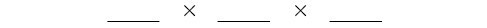There are four options for the first place, so we write a 4 on the first line.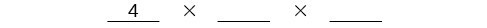After the first place has been filled, there are three options for the second place so we write a 3 on the second line.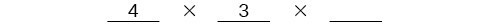After the second place has been filled, there are two options for the third place so we write a 2 on the third line. Finally, we find the product.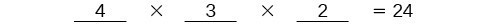There are 24 possible permutations of the paintings.

### How To: Given $n$ distinct options, determine how many permutations there are.

1. Determine how many options there are for the first situation.
2. Determine how many options are left for the second situation.
3. Continue until all of the spots are filled.
4. Multiply the numbers together.

### Example 2: Finding the Number of Permutations Using the Multiplication Principle

At a swimming competition, nine swimmers compete in a race.

1. How many ways can they place first, second, and third?
2. How many ways can they place first, second, and third if a swimmer named Ariel wins first place? (Assume there is only one contestant named Ariel.)
3. How many ways can all nine swimmers line up for a photo?

### Solution

1. Draw lines for each place.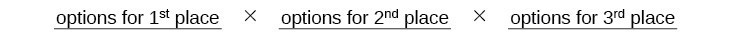There are 9 options for first place. Once someone has won first place, there are 8 remaining options for second place. Once first and second place have been won, there are 7 remaining options for third place.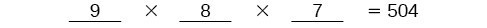Multiply to find that there are 504 ways for the swimmers to place.

2. Draw lines for describing each place.We know Ariel must win first place, so there is only 1 option for first place. There are 8 remaining options for second place, and then 7 remaining options for third place.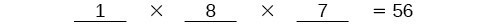Multiply to find that there are 56 ways for the swimmers to place if Ariel wins first.

3. Draw lines for describing each place in the photo.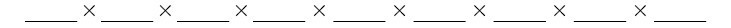There are 9 choices for the first spot, then 8 for the second, 7 for the third, 6 for the fourth, and so on until only 1 person remains for the last spot.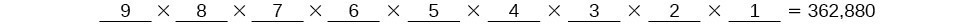There are 362,880 possible permutations for the swimmers to line up.

### Analysis of the Solution

Note that in part c, we found there were 9! ways for 9 people to line up. The number of permutations of $n$ distinct objects can always be found by $n!$.

A family of five is having portraits taken. Use the Multiplication Principle to find the following.

### Try It 3

How many ways can the family line up for the portrait?

Solution

### Try It 4

How many ways can the photographer line up 3 family members?

Solution

### Try It 5

How many ways can the family line up for the portrait if the parents are required to stand on each end?

Solution

## Finding the Number of Permutations of n Distinct Objects Using a Formula

For some permutation problems, it is inconvenient to use the Multiplication Principle because there are so many numbers to multiply. Fortunately, we can solve these problems using a formula. Before we learn the formula, let’s look at two common notations for permutations. If we have a set of $n$ objects and we want to choose $r$ objects from the set in order, we write $P\left(n,r\right)$. Another way to write this is ${n}_{}{P}_{r}$, a notation commonly seen on computers and calculators. To calculate $P\left(n,r\right)$, we begin by finding $n!$, the number of ways to line up all $n$ objects. We then divide by $\left(n-r\right)!$ to cancel out the $\left(n-r\right)$ items that we do not wish to line up.

Let’s see how this works with a simple example. Imagine a club of six people. They need to elect a president, a vice president, and a treasurer. Six people can be elected president, any one of the five remaining people can be elected vice president, and any of the remaining four people could be elected treasurer. The number of ways this may be done is $6\times 5\times 4=120$. Using factorials, we get the same result.

$\frac{6!}{3!}=\frac{6\cdot 5\cdot 4\cdot 3!}{3!}=6\cdot 5\cdot 4=120$

There are 120 ways to select 3 officers in order from a club with 6 members. We refer to this as a permutation of 6 taken 3 at a time. The general formula is as follows.

$P\left(n,r\right)=\frac{n!}{\left(n-r\right)!}$

Note that the formula stills works if we are choosing all $n$ objects and placing them in order. In that case we would be dividing by $\left(n-n\right)!$ or $0!$, which we said earlier is equal to 1. So the number of permutations of $n$ objects taken $n$ at a time is $\frac{n!}{1}$ or just $n!\text{.}$

### A General Note: Formula for Permutations of n Distinct Objects

Given $n$ distinct objects, the number of ways to select $r$ objects from the set in order is

$P\left(n,r\right)=\frac{n!}{\left(n-r\right)!}$

### How To: Given a word problem, evaluate the possible permutations.

1. Identify $n$ from the given information.
2. Identify $r$ from the given information.
3. Replace $n$ and $r$ in the formula with the given values.
4. Evaluate.

### Example 3: Finding the Number of Permutations Using the Formula

A professor is creating an exam of 9 questions from a test bank of 12 questions. How many ways can she select and arrange the questions?

### Solution

Substitute $n=12$ and $r=9$ into the permutation formula and simplify.

$\begin{array}{l}\text{ }P\left(n,r\right)=\frac{n!}{\left(n-r\right)!}\hfill \\ P\left(12,9\right)=\frac{12!}{\left(12 - 9\right)!}=\frac{12!}{3!}=79\text{,}833\text{,}600\hfill \end{array}$

There are 79,833,600 possible permutations of exam questions!

### Analysis of the Solution

We can also use a calculator to find permutations. For this problem, we would enter 15, press the ${}_{n}{P}_{r}$ function, enter 12, and then press the equal sign. The ${}_{n}{P}_{r}$ function may be located under the MATH menu with probability commands.

### Could we have solved  using the Multiplication Principle?

Yes. We could have multiplied $15\cdot 14\cdot 13\cdot 12\cdot 11\cdot 10\cdot 9\cdot 8\cdot 7\cdot 6\cdot 5\cdot 4$ to find the same answer.

A play has a cast of 7 actors preparing to make their curtain call. Use the permutation formula to find the following.

### Try It 6

How many ways can the 7 actors line up?

Solution

### Try It 7

How many ways can 5 of the 7 actors be chosen to line up?

Solution